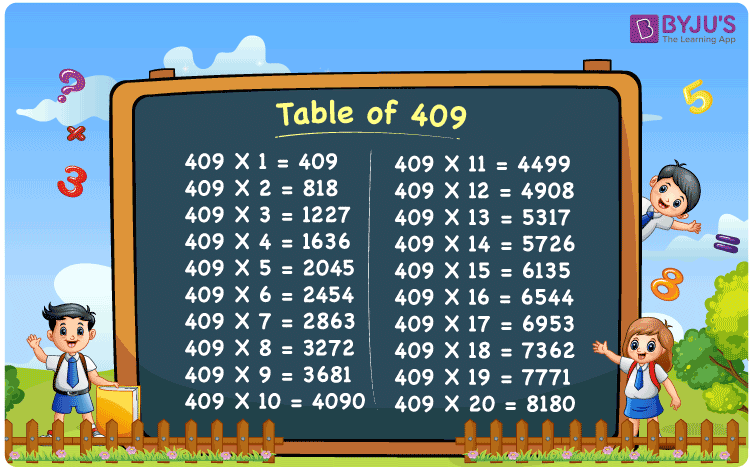Checkout JEE MAINS 2022 Question Paper Analysis : Checkout JEE MAINS 2022 Question Paper Analysis :

# Table of 409

The Table of 409 is the multiplication table that includes the multiples when 409 is multiplied by consecutive natural numbers. 409 times table represents the multiplication of 409 by a number on repeated addition form. For example, 409 x 3 = 409 + 409 + 409 = 1227. Multiplication tables help us to solve mathematical problems quickly. Download the PDF of table 409 at BYJU’S for free.

## Table of 409 Chart## What is 409 Times Table?

The table below shows the repeated addition of the number 409 to get the required multiple.

 409×1 = 409 409 409×2 = 818 409 + 409 = 818 409×3 = 1227 409 + 409 + 409 = 1227 409×4 = 1636 409 + 409 + 409 + 409 = 1636 409×5 = 2045 409 + 409 + 409 + 409 + 409 = 2045 409×6 = 2454 409 + 409 + 409 + 409 + 409 + 409 = 2454 409×7 = 2863 409 + 409 + 409 + 409 + 409 + 409 + 409 = 2863 409×8 = 3272 409 + 409 + 409 + 409 + 409 + 409 + 409 + 409 = 3272 409×9 = 3681 409 + 409 + 409 + 409 + 409 + 409 + 409 + 409 + 409 = 3681 409×10 = 4090 409 + 409 + 409 + 409 + 409 + 409 + 409 + 409 + 409 + 409 = 4090

## Multiplication Table of 409

Here is the table of 409 up to 20 times below.

 409 × 1 = 409 409 × 2 = 818 409 × 3 = 1227 409 × 4 = 1636 409 × 5 = 2045 409 × 6 = 2454 409 × 7 = 2863 409 × 8 = 3272 409 × 9 = 3681 409 × 10 = 4090 409 × 11 = 4499 409 × 12 = 4908 409 × 13 = 5317 409 × 14 = 5726 409 × 15 = 6135 409 × 16 = 6544 409 × 17 = 6953 409 × 18 = 7362 409 × 19 = 7771 409 × 20 = 8180

## Solved Example on Table of 409

Evaluate: 409 x 5 + 1210

Solution: Given,

409 x 5 + 1210

From the table of 409, we have;

409 x 5 = 2045

Therefore,

2045 + 1210 = 3255

## Frequently Asked Questions on Table of 409

### What is table of 409 in Maths?

In Maths, the multiplication table of 409 represents multiples of 409, such as:

409 x 1 = 409

409 x 2 = 818

409 x 3 = 1227

409 x 4 = 1636

409 x 5 = 2045

### What is the value of 409 times 11?

409 times 11 is equal to 4499.

### Is 409 a prime number?

Yes, 409 is a prime number, that has only two factors, i.e., 1 and 409.